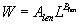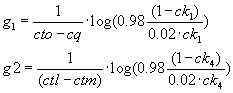# Growth of salmonids: eggs and fry

### Fry Growth Help: Parameters for bioenergetic modeling of fry growth

#### Weight/Length relationship: Alen, Blen

These parameters are regression parameters for juvenile Chinook salmon relating Weight to Length where:#### Bioenergetics Parameters

The other parameters and algorithm used in the fry growth model is based on Chinook bioenergetic parameters as published by UW Sea Grant Institute, 214 Goodnight Hall, 1975 Willow Drive, Madison, WI 53706-1177 http://www.seagrant.wisc.edu.

Fish bioenergetics technical support is available at bioenergetics web page. The growth of a fish is defined with the following:where C = consumption, R = respiration, D = SDA, U = excretion,F = egestion, W = weight, V= velocity.

ca = .303
cb = -0.275
cq = 5
cto = 15
ctm = 18
ctl = 24
ck1 = 0.36
ck4 = 0.01ka = (.36 * l1) / (1+.36(l1-1))
kb = (.01*l2)/(1+.01(l2-1))
ra = .00264
rb = -0.217
rq = 0.06818
rto = 0.0234
rtm = 0
rtl = 25
rk1 = 27.5
rk4 = 0.13
act = 9.7
bact = 0.405
sda = 0.172
fa = 0.212
fb = -0.222
fg = 0.631
ua = 0.0314
ub = 0.58
ug = -0.299
alpha = 5764
beta = 0.9862
dy = 5250

The user selects dr (prey energy density) and
p (proportion of maximum consumption)

We assume no differences in bioenergetics of juvenile and adult chinook once the fish become fry and have absorbed their yolk sac completely. Although is is possible that the fish can begin eating prior to this point, the overall contribution to growth is small.

Growth in the river is terminated by one of three methods:

• The fish reaches a certain size (and migrates)
• A certain day of the year marks the end of growth (could be day 365, of course)
• The river temperatures reach a certain value (and fish stop feeding). This assumes rising temperatures through the spring and summer and attainment of the temperature in the system, otherwise it will grow to the end of the year.